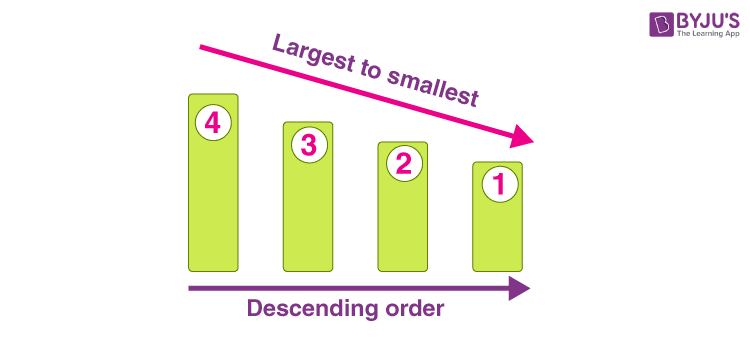Checkout JEE MAINS 2022 Question Paper Analysis : Checkout JEE MAINS 2022 Question Paper Analysis :

# Descending Order

In Mathematics, if a pattern of numbers is in the decreasing value, then the numbers are in descending order. It means that the numbers are arranged in the highest order to the lowest order. In this article, we will learn the meaning of descending order, the symbol used to represent the decreasing order, and many solved examples.

## Descending Order Meaning

If the information is sorted from highest to lowest, it is said to be in descending order. For example 10, 9, 8, 7, 6, 5, 4, 3, 2, 1 are arranged in descending order. In other words, if the numbers are arranged from the largest to the smallest number, it is said to be in descending order.

In simple words, descending order is defined as an arrangement in the highest to lowest format. These concepts are related to decimals, numbers, fractions or amount of money.

Example of Descending Order

24, 20, 18, 12, 7 are arranged in descending order.

This is also known as decreasing order of numbers.

## Descending Order Symbol

The symbol used to represent the order in descending form is ‘ > ‘. It shows the given sequence in increasing to decreasing order. Example: numbers from 1 to 10 can be represented in this form using the descending symbol. 10 > 9 > 8 > 7 > 6  > 5 > 4 > 3 > 2 >1.

## Descending Order Alphabet

As we know descending order means to go from biggest to smallest. In alphabets from A to Z, the descending order will be from Z to A.

Therefore, the descending order of alphabets (when we assign the numerical value for alphabets) can be written as

Z, Y, X, W, V, U, T, S, R, Q, P, O, N, M, L, K, J, I, H, G, F, E, D, C, B, A.

You could be asked to arrange numbers, be it whole, real, fractions or decimals in descending or ascending order. Below are some example questions from the descending order concept which will help you to get through with this concept.### Descending Order Examples

Example 1: Arrange the given numbers in descending order:
99, 101, 54, 87, 49, 34, 107, 89, 09, 16

Solution:

To arrange the given numbers in descending order, place them from largest to the smallest number as shown below:
107, 101, 99, 89, 87, 54, 49, 34, 16, 09

Example 2: Arrange the given numbers in descending order:
32 , 52 , 42 , 62

Solution:

• 32 = 3 x 3 = 9
• 52 = 5 x 5 = 25
• 24 = 2 x 2 x 2 x 2 = 16
• 62 = 6 x 6 = 36

The numbers are arranged in descending order

36, 25, 16, 9

62 > 52 > 42 > 32

Example 3: Five kids empties their piggy bank. These are the amounts of coins each one had in their piggy banks :
Rs. 110, Rs. 450, Rs. 50, Rs. 80, Rs. 76

Write this amount of money in descending order.

Solution:

The amount of money in from highest to lower order is:

Rs. 450, Rs. 110, Rs. 80, Rs. 76, Rs. 50

Example 4: List the following dates in descending 0rder.

3rd Jan 2018, 7th May 2018, 1st Jan 2018

Solution:

The descending order dates for the given list are:

7th May 2018, 3rd Jan 2018 1st Jan 2018

Example 5: Arrange the following numbers in descending order?
9, 8, 2, 7, 3, 6, 4, 5, 11, 90, 32, 56, 78, 34, 76, 23, 65, 1, 54, 98, 53, 30

Solution :

The numbers arranged in descending order for the given data:

98, 90, 78, 76, 65, 56, 54, 53, 34, 32, 30, 23, 11, 9, 8, 7, 6, 5, 4, 3, 2, 1

 Related Links Decimals Integers Number system Fractions

## Frequently Asked Questions on Descending Order

### What is increasing and decreasing order?

If the set of numbers are written from smallest to largest, then the numbers are said to be in increasing order, whereas when these numbers are written from largest to smallest, then it is called decreasing order.

### What is the meaning of descending?

Descending means moving towards down, small, or least from top, big or large. For example, descend from the top of a hill.

### What is the meaning of increasing order?

Increasing order means the arrangement of numbers from smallest to largest. For example, the increasing order of 56, 29, 84, 60, 45 is 29, 45, 56, 60, 84.

### What is the sign of decreasing order?

The symbol used to represent the order in descending form is ‘ > ‘. Example: numbers from 10 to 15 can be represented in this form using the descending symbol as 15 > 14 > 13 > 12 > 11 > 10.

### How do you arrange in increasing order?

We can arrange the numbers in increasing order using the symbol “<” (less than). For example, the numbers 20, 40, 10, 35, 50 can be written in the increasing order as 10 < 20 < 35 < 40 < 50.
Test your knowledge on Descending Order